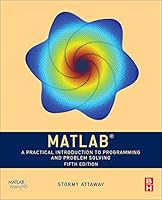# MATLAB: A Practical Introduction to Programming and Problem Solving## Book Description

MATLAB: A Practical Introduction to Programming and Problem Solving, winner of TAA’s 2017 Textbook Excellence Award ("Texty"), guides the reader through both programming and built-in to easily exploit MATLAB's extensive capabilities for tackling and scientific problems. Assuming no knowledge of programming, this book starts with programming concepts, such as variables, assignments, and selection statements, moves on to loops, and then solves problems using both the programming concept and the power of MATLAB. The fifth edition has been updated to reflect the functionality of the current version of MATLAB (R2018a), including the addition of local in scripts, the new string type, coverage of recently introduced to import data from web sites, and updates to the Live Editor and App Designer.

• Presents programming concepts and MATLAB built-in functions side by side, giving students the ability to program efficiently and exploit the power of MATLAB to solve technical problems
• Offers sections on common pitfalls and programming guidelines that direct students to best practice procedures
• Tests conceptual understanding of the material with Quick Questions! and Practice sections within each chapter

NEW TO THE FIFTH EDITION

• Use of MATLAB Version R2018a
• A revised Text Manipulation chapter, which includes manipulating character vectors as well as the new string type
• Introduction to alternate MATLAB platforms, including MATLAB Mobile
• Local functions within scripts
• The new output format for most expression types
• Introduction to the RESTFUL web functions which import data from web sites
• Increased coverage of App Designer
• Introduction to recording from a built-in device such as a microphone
• Modified and new end-of-chapter exercises
• More coverage of data structures including categorical arrays and tables
• Increased coverage of built-in functions in MATLAB

Part 1: Introduction to Programming Using MATLAB
Chapter 1: Introduction to MATLAB
Chapter 2: Vectors and Matrices
Chapter 3: Introduction to MATLAB Programming
Chapter 4: Selection Statements
Chapter 5: Loop Statements and Vectorizing
Chapter 6: MATLAB Programs
Chapter 7: Text Manipulation
Chapter 8: Data Structures
Part 2: Advanced Topics for Problem Solving with MATLAB
Chapter 9: Data Transfer
Chapter 11: Introduction to Object-Oriented Programming and